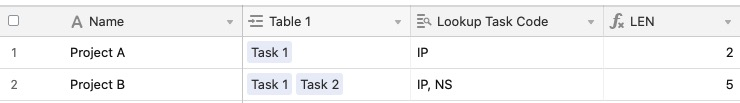• English
• Account
• API
• Integrations
• Enterprise
• Resources
Extracting text from a field
• 29 Nov 2022
• Dark
Light

# Extracting text from a field

• Dark
Light

Article Summary

This article will cover how to extract specified values (and amounts) from fields. There are four different functions that can help you do that:

• `LEFT`
• `RIGHT`
• `LEN`
• `MID`

## LEN()

This is a very straightforward function that simply returns the length of a string. You can reference a value / field / string within the function following this format: `LEN(string).`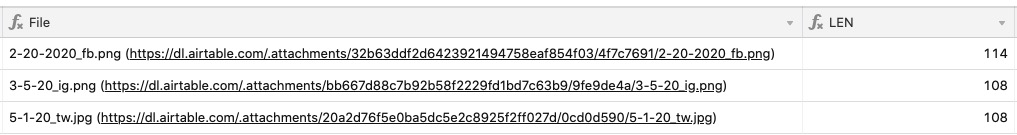``````LEN  ({File})
``````

A popular use for this function is determining word and character counts in order to alert you if you've ran over a specified limit (think character counts for tweets, word counts for blog articles).

Counting characters

Let's say that you have a base setup to track social media content, and want to make sure your tweets don't exceed the 280 character limit. You can use a formula like the following to output the character count of the text field:

``````LEN  ({Long Text})
``````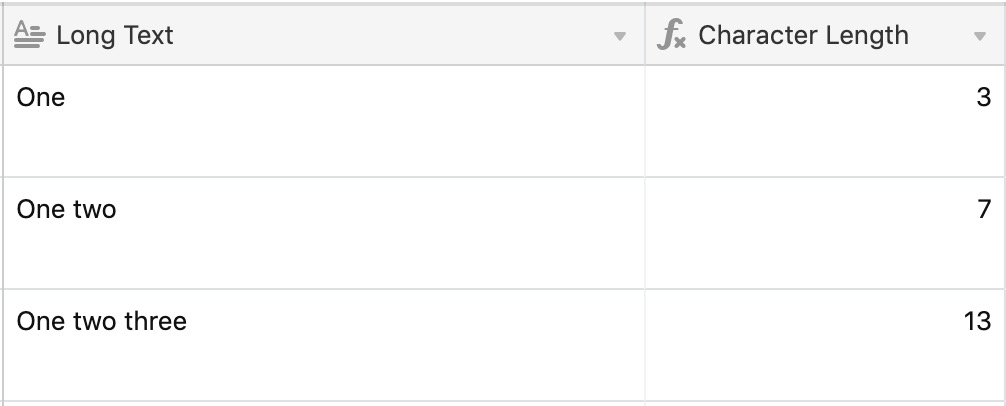Displaying a message based on character count

Now, let's say that you're not so interested in the exact character count, but more so that you're under the 280 character limit. Here's an example of an IF statement you can write to check for this and output a message depending on the character count:

``````IF(LEN ({Long Text})>280, "❌Over Limit" , "✅Under Limit" )
``````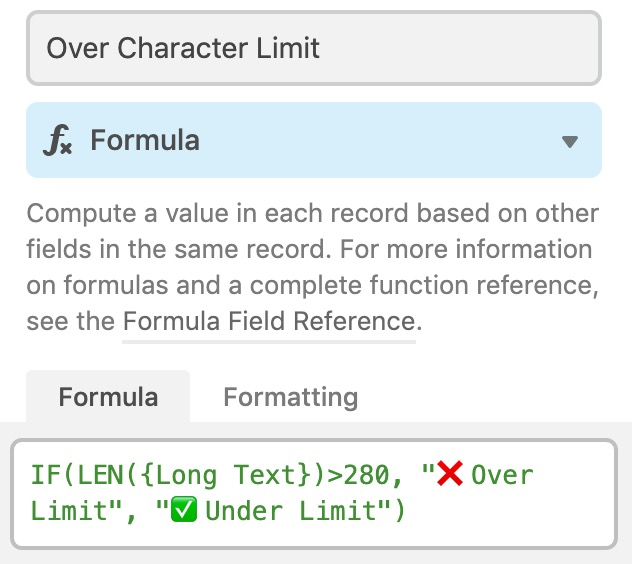Using this formula, you'll see either ❌Over Limit or ✅Under Limit for each record depending on the character count in the field your formula is watching.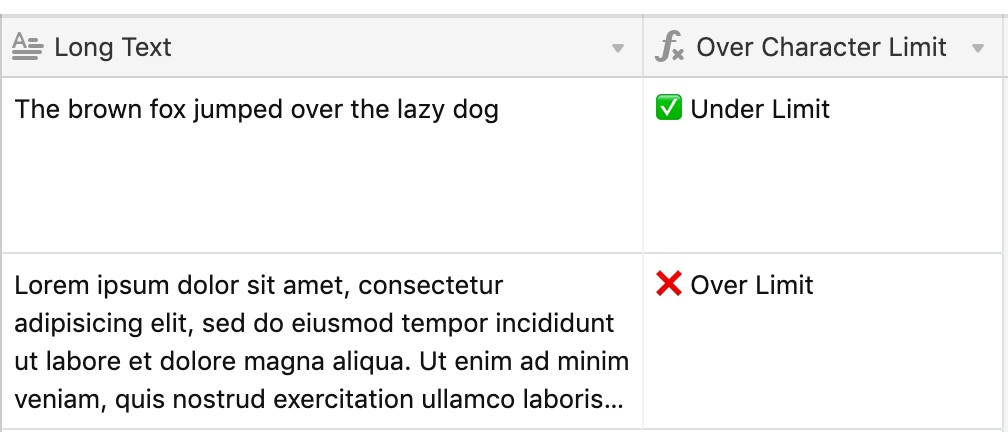Counting words

For a different workflow, you may be writing editorial content and want to ensure your articles don't exceed a certain word count. You can use a similar formula to count the number of spaces in a text field, which in turn will give you a total count of words.

``````IF({Text Field}=BLANK(), 0, LEN({Text Field}) - LEN(SUBSTITUTE({Text Field}, ' ', ''))+1)
``````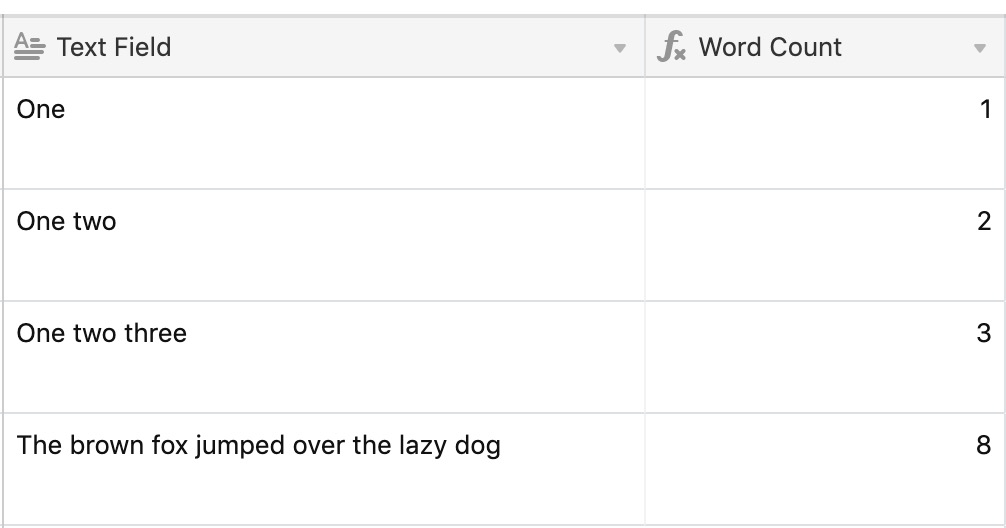## LEFT() and RIGHT()

The `LEFT()` and `RIGHT()` functions work similarly to one another by extracting a certain number of characters from the beginning (left) or end (right) of a string.

• `LEFT("quick brown fox", 5)` would result in "Quick"
• `RIGHT("quick brown fox", 3)` would result in "Fox"

These are helpful functions when to use when you need to extract data from a number of records that share a similar structure. For example, you may be importing a dataset where a single cell contains both a date string and a text string, like `02-25-2018_In-Progress`.

Assuming that the dates follow the same format of DD-MM-YYYY, you can use LEFT() to extract the date from the full field value:

``````LEFT  ({Field Name},  10  )
``````

## MID()

This function lets you extract a certain number of characters starting at a specific place within a string. It follows this format: `MID(string, whereToStart, count)`. Let's use the example field value from the previous section to demonstrate.

For a field that contains this value, `02-25-2018_In-Progress`, we might want to only extract the status portion of the string. Since the dates always follow the same format, and the underscore character is always the 11th character in the string, we want to extract any text past that ( starting at the 12th character) to obtain the status.

``````MID  ({Field Name},  12  ,  30  )
``````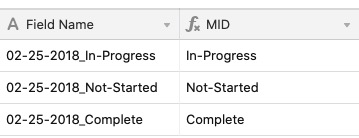The 30 specified at the end is the count of characters I want to extract. This is a number of characters set to account for the different statuses that may be present in the dataset (e.g. Not Started, Complete). You can always set an arbitrarily high number of characters (like 100, 500, 1000, etc) to account for variations in the dataset.

## Working with arrays

When working with rollup or lookup fields, the value that is returned is formatted as an array. An array can contain a list of values, rather than a single value, and is treated differently than a plain string of text (even if that string had multiple values).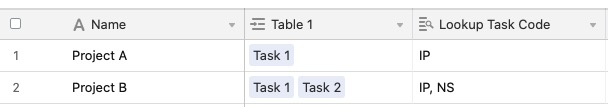When using arrays with any of the functions covered in this article, you'll run into an error if you just try to reference the array.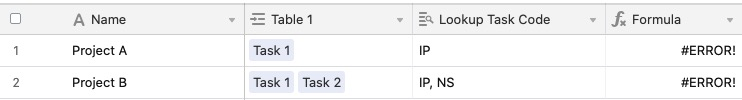You'll need to join the items in the array into a string in order to use these functions. The adjusted formula `LEN(ARRAYJOIN({Lookup Task Code}))` will convert the arrayed results on the lookup into a readable string, and then return the length of that string.